# Olympiad Test: Rational Numbers - 2

## 10 Questions MCQ Test Mathematical Olympiad Class 8 | Olympiad Test: Rational Numbers - 2

Description
Attempt Olympiad Test: Rational Numbers - 2 | 10 questions in 20 minutes | Mock test for Class 8 preparation | Free important questions MCQ to study Mathematical Olympiad Class 8 for Class 8 Exam | Download free PDF with solutions
QUESTION: 1

Solution:
QUESTION: 2

Solution:
QUESTION: 3

### Which number is largest among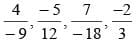Solution:
QUESTION: 4

Which number is smallest among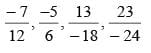Solution:

LCM of 12, 6, 18, 24 = 72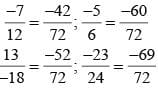QUESTION: 5

Closure property of rational number will be value in:

Solution:

Closure property of rational numbers is satisfied for addition, subtraction and multiplication.
Closure property : The sum of two rational numbers will be a rational of addition for rational number.

QUESTION: 6

The difference between two numbers is 22 and their product is 240, what is the difference between their reciprocals ?

Solution:

p – q = 22, pq = 240.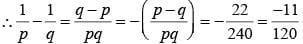QUESTION: 7

If a : b = 2 : 3, then what will be the value of  ab a+b/a-b?

Solution:

Here a+b/a-b Dividing Numerator andDenominator by b, we have,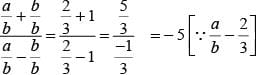QUESTION: 8

If a : b = 1:2, then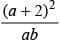=

Solution: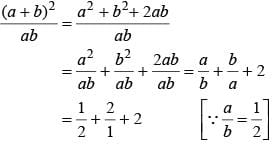= 9/2

QUESTION: 9

The sum of two numbers ‘p’ and ‘q’ is 16 and their product is 48, then,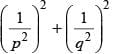Solution: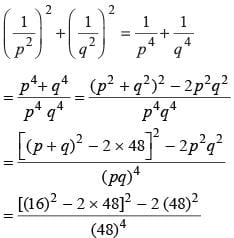= 8.24 x 10-5

QUESTION: 10

The sum of two numbers is 24 and their difference is 10, then,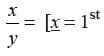number, y = 2nd number].

Solution:

Solution :- x + y = 24

The difference between x and y is 10. In other words, x minus y equals 10 and can be written as equation B:

x - y = 10

Now solve equation B for x to get the revised equation B:

x - y = 10

x = 10 + y

Then substitute x in equation A from the revised equation B and then solve for y:

x + y = 24

10 + y + y = 24

10 + 2y = 24

2y = 14

y = 7

Now we know y is 7. Which means that we can substitute y for 7 in equation A and solve for x:

x + y = 24

x + 7 = 24

x = 17

So x/y = 17/7Use Code STAYHOME200 and get INR 200 additional OFF Use Coupon Code